# paretoplot

Pareto plot of multiobjective values

Since R2022a

## Syntax

``paretoplot(val)``
``paretoplot(val,objlabels)``
``paretoplot(val,objindex)``
``h = paretoplot(___)``
``paretoplot(ax,___)``

## Description

example

````paretoplot(val)` creates a Pareto plot of the objectives in `val`. If `val` contains more than three objectives, `paretoplot` plots the first three objectives.```

example

````paretoplot(val,objlabels)` creates a Pareto plot of the objectives listed in `objlabels`. Use this syntax if you have separate labels for each objective function.```

example

````paretoplot(val,objindex)` creates a Pareto plot of the objectives listed in `objindex`. Use this syntax is your objective functions are not labeled.```

example

````h = paretoplot(___)`, for any previous input syntax, returns a handle `h` to the resulting scatter object. Use `h` to set properties of the scatter object after creation.```
````paretoplot(ax,___)` plots into the axes with handle `ax`.```

## Examples

collapse all

Create and solve a multiobjective optimization problem using optimization variables.

```x = optimvar("x",LowerBound=-1,UpperBound=2); prob = optimproblem; prob.Objective.obj1 = x^2; prob.Objective.obj2 = (x-1)^2; [sol,fval] = solve(prob,Solver="paretosearch");```
```Solving problem using paretosearch. Pareto set found that satisfies the constraints. Optimization completed because the relative change in the volume of the Pareto set is less than 'options.ParetoSetChangeTolerance' and constraints are satisfied to within 'options.ConstraintTolerance'. ```

Plot the Pareto front.

`paretoplot(sol)`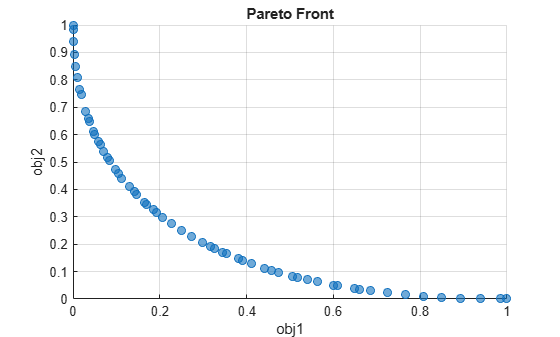Create and solve a multiobjective optimization problem with four named objectives.

```x = optimvar("x",2,LowerBound=-2,UpperBound=4); prob = optimproblem; prob.Objective.obj1 = norm(x)^2; prob.Objective.obj2 = norm(x - [1;0])^2; prob.Objective.obj3 = norm(x - [0;1])^2; prob.Objective.obj4 = norm(x - [1;1])^2; sol = solve(prob,Solver="paretosearch");```
```Solving problem using paretosearch. Pareto set found that satisfies the constraints. Optimization completed because the relative change in the distance of the Pareto set is less than 'options.ParetoSetChangeTolerance' and constraints are satisfied to within 'options.ConstraintTolerance'. ```

Create a Pareto plot of the first three objectives;

`paretoplot(sol)`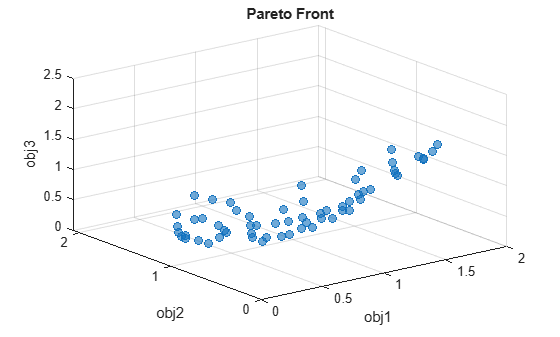Create a Pareto plot of the last three objectives.

`paretoplot(sol,["obj2" "obj3" "obj4"])`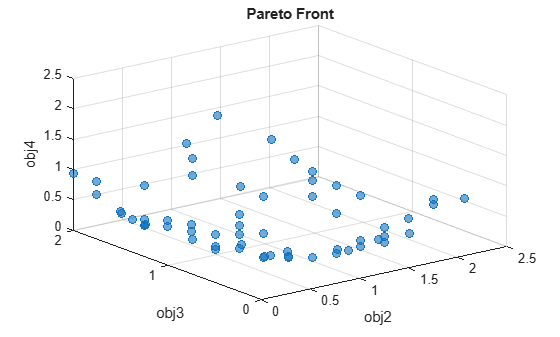Create and solve a multiobjective optimization problem with four objectives. The objective function returns a four-element vector.

```x = optimvar("x",2,LowerBound=-2,UpperBound=4); prob = optimproblem; obj = [norm(x)^2,norm(x - [1;0])^2,norm(x - [0;1])^2,norm(x - [1;1])^2]; prob.Objective = obj; sol = solve(prob,Solver="paretosearch");```
```Solving problem using paretosearch. Pareto set found that satisfies the constraints. Optimization completed because the relative change in the distance of the Pareto set is less than 'options.ParetoSetChangeTolerance' and constraints are satisfied to within 'options.ConstraintTolerance'. ```

Create a Pareto plot of the first three objectives;

`paretoplot(sol)`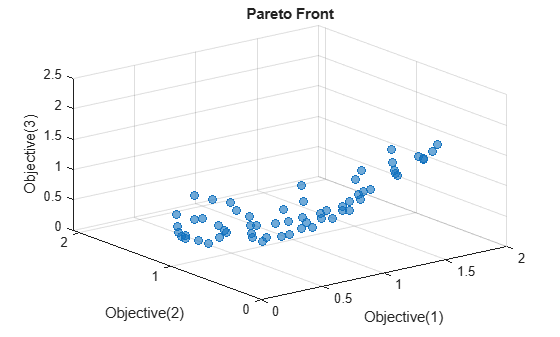Create a Pareto plot of the last three objectives.

`paretoplot(sol,[2 3 4])`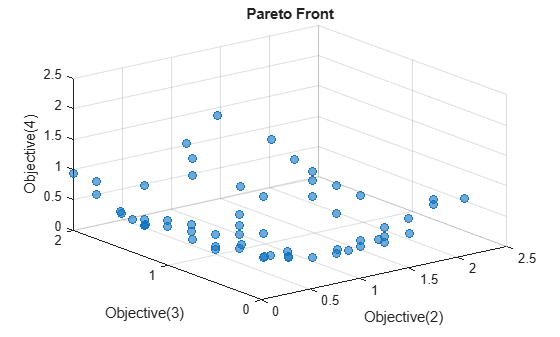Create and solve a multiobjective optimization problem using optimization variables.

```x = optimvar("x",LowerBound=-1,UpperBound=2); prob = optimproblem; prob.Objective.obj1 = x^2; prob.Objective.obj2 = (x-1)^2; [sol,fval] = solve(prob,Solver="paretosearch");```
```Solving problem using paretosearch. Pareto set found that satisfies the constraints. Optimization completed because the relative change in the volume of the Pareto set is less than 'options.ParetoSetChangeTolerance' and constraints are satisfied to within 'options.ConstraintTolerance'. ```

Plot the Pareto front. To enable editing, obtain a handle to the plot.

`h = paretoplot(sol);`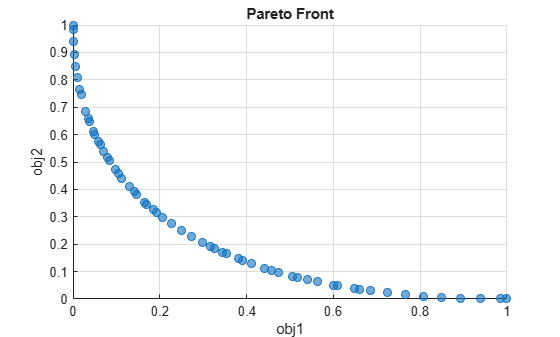Change the markers from blue `'o'` to red `'x'`. To obtain an undistorted view, set the axes to have equal lengths.

```h.Marker = "x"; h.MarkerEdgeColor = "r"; axis equal```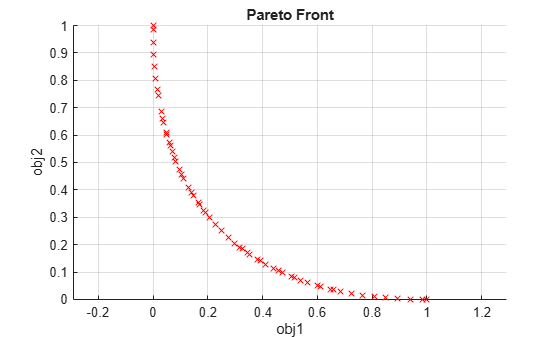For a complete list of editable properties, see Scatter Properties.

## Input Arguments

collapse all

Optimization values, specified as an `OptimizationValues` object. Typically, `vals` is the solution to a multiobjective problem, the `sol` output of `solve`.

`paretoplot` can plot either two or three objectives. If you have more than three objectives, `paretoplot` plots the first three. Specify the two or three objectives to plot using the `objlabels` or `objindex` arguments.

Example: `sol`

Objective function labels, specified as a string vector of two or three entries. The entries are objective function labels in the optimization problem.

Example: `["obj1" "obj2"]`

Data Types: `char` | `string`

Objective function indices, specified as a positive integer vector. `objindex` must contain two or three entries in the range from 1 through the number of objectives.

Example: `[4 1 3]`

Data Types: `double`

Axes for plot, specified as a handle.

## Version History

Introduced in R2022a